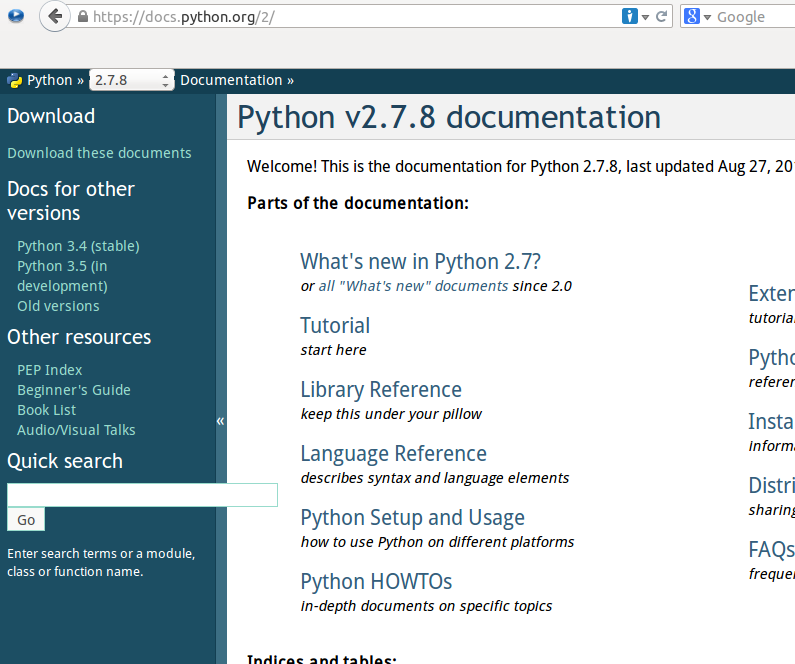# Python文档

1.文档是一种对软件系统的书面描述； 2.文档应当精确地描述软件系统； 3.软件文档是软件工程师之间用作沟通交流的一种方式； 4.文档的类型有很多种，包括软件需求文档，设计文档，测试文档，用户手册等； 5.文档的呈现方式有很多种，可以是传统的书面文字形式或图表形式，也可是动态的网页形式

## 查看python文档

python文档的网址：https://docs.python.org/2/，这是python2.x，从这里也可以找到python3.x的文档。``````>>> dir(list)
['__add__', '__class__', '__contains__', '__delattr__', '__delitem__', '__delslice__', '__doc__', '__eq__', '__format__', '__ge__', '__getattribute__', '__getitem__', '__getslice__', '__gt__', '__hash__', '__iadd__', '__imul__', '__init__', '__iter__', '__le__', '__len__', '__lt__', '__mul__', '__ne__', '__new__', '__reduce__', '__reduce_ex__', '__repr__', '__reversed__', '__rmul__', '__setattr__', '__setitem__', '__setslice__', '__sizeof__', '__str__', '__subclasshook__', 'append', 'count', 'extend', 'index', 'insert', 'pop', 'remove', 'reverse', 'sort']

>>> help(list.__mul__)

Help on wrapper_descriptor:

__mul__(...)
x.__mul__(n) <==> x*n
``````

``````>>> print(list.__mul__.__doc__)     #与help(list.__mul__)显示的内容一致
x.__mul__(n) <==> x*n

>>> print(list.index.__doc__)       #查看index的文档
L.index(value, [start, [stop]]) -> integer -- return first index of value.
Raises ValueError if the value is not present.
``````

## 给自己的程序加上文档

``````>>> def qiwsir():
...     """I like python"""
...     print "http://qiwsir.github.io"
...
>>> qiwsir()
http://qiwsir.github.io

>>> print(qiwsir.__doc__)   #用这种方法可以看自己写的函数中的文档
I like python

>>> help(qiwsir)            #其实就是调用__doc__显示的内容

Help on function qiwsir in module __main__:

qiwsir()
I like python
``````

``````#!/usr/bin/env python
#coding:utf-8

import random

number = random.randint(1,100)

guess = 0

while True:

num_input = raw_input("please input one integer that is in 1 to 100:")
guess +=1

if not num_input.isdigit():
elif int(num_input)<0 and int(num_input)>=100:
print "The number should be in 1 to 100."
else:
if number==int(num_input):
print "OK, you are good.It is only %d, then you successed."%guess
break
elif number>int(num_input):
print "your number is more less."
elif number<int(num_input):
else:
print "There is something bad, I will not work"
``````

``````"""
This is a game.
I am Qiwei.
I like python.
I am writing python articles in my website.
My website is http://qiwsir.github.io
You can learn python free in it.
"""

#!/usr/bin/env python
#coding:utf-8

import random

number = random.randint(1,100)

guess = 0

while True:

num_input = raw_input("please input one integer that is in 1 to 100:")
guess +=1

if not num_input.isdigit():
elif int(num_input)<0 and int(num_input)>=100:
print "The number should be in 1 to 100."
else:
if number==int(num_input):
print "OK, you are good.It is only %d, then you successed."%guess
break
elif number>int(num_input):
print "your number is more less."
elif number<int(num_input):